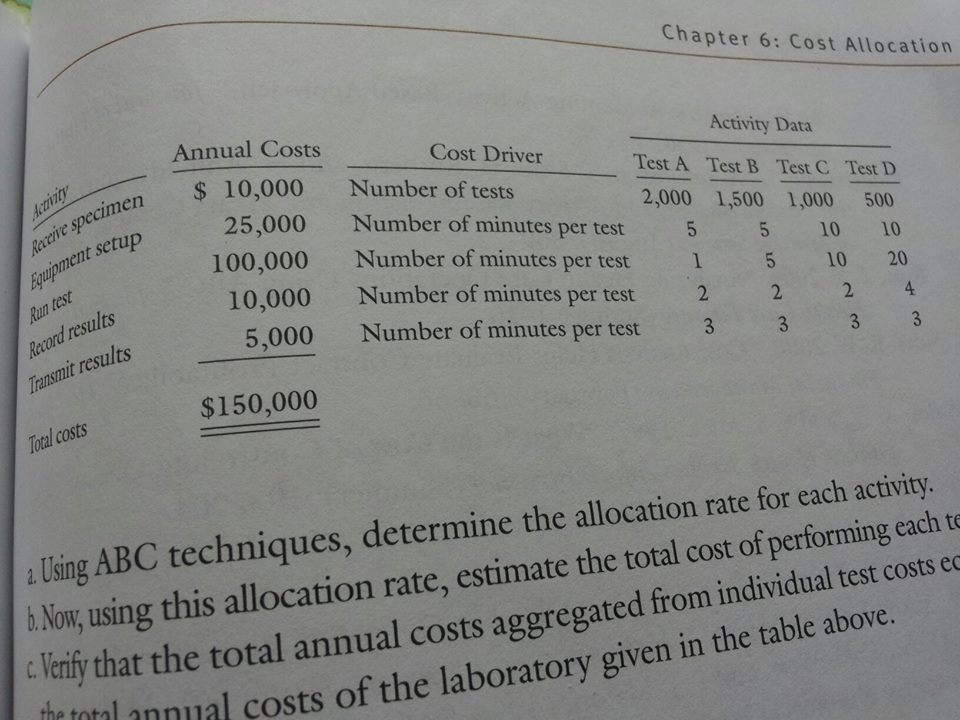### Create an Account

Home / Questions / USING ABC techniques determine the allocation rate for each activityB now using this all...

# USING ABC techniques determine the allocation rate for each activityB now using this allocation rate estimate thetotal cost of performing each test c verify that the total annual costs

USING ABC techniques , determine the allocation rate for each activity.B) now , using this allocation rate , estimate thetotal cost of performing each test. c) verify that the total annual costs aggregated from individual test costs equal the total annual costs of the laborator given in the tableMay 14 2020 View more View LessSubscribe To Get Solution